# Units - math word problems

#### Number of problems found: 3146

• Jar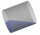From the cylinder shaped jar after tilting spilled water so that the bottom of the jar reaches the water level accurately into half of the base. Height of jar h = 7 cm and a jar diameter D is 12 cm. How to calculate how much water remains in the jar?
• Apartment areaAnton wanted to measure the area of the apartment. But he bought a meter only 1.5m long. Later he recalled that he had two meters long at 4.5m and 18m at home. How many times are home gauges longer than the meter he bought?
• Two machinesThe first machine can produce parts in 24 hours, the second in 16 hours. The first started working in the morning at 6:00 pm. Second at 10:00 o'clock. At what hour were all parts made?
• Microsaving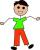Honza saved 72 crowns. Pepík saved 9 crowns more than Honza. How many crowns saved Pepík?
• The cyclistThe cyclist drove from A to B, 60 km away. The first half drove at 30km/h, the second at 20km/h. How long did it take for him?
• Pyramid four sidesIn a regular tetrahedral pyramid is a body height 38 cm and a wall height 42 cm. Calculate the surface area of the pyramid; the result round to square centimeters.
• A rectangular patioA rectangular patio measures 20 ft by 30 ft. By adding x feet to the width and x feet to the length, the area is doubled. Find the new dimensions of the patio.
• Welders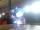Welder do the job for six hours, the second one for 8 hours. How long do the job third welder if all together can do job in 2 hours?
• School 6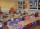Jirka lives 600m from the school, Honzík 1200m. How many kilometers a week does Honzik go over Jirka on his way to school and back? How many times more Honzík will go than Jirka?
• Angle of the body diagonalsUsing vector dot product calculate the angle of the body diagonals of the cube.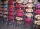Cris had 15000 . He spent 1/3 of his money on a table and the remaining on 5 similar chairs that cost the same. How much did one chair cost?
• Cuboid wallsIf the areas of three adjacent faces of a cuboid are 8 cm², 18 cm² and 25 cm². Find the volume of the cuboid.
• Right pyramid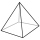A right pyramid on a base 4 cm square has a slant edge of 6 cm. Calculate the volume of the pyramid.
• Bronze, tin and copper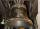Bronze is an alloy of tin and copper. An alloy of 10% tin and 90% copper is Gunmetal. If it contains 20% tin and 80% copper, it is bell metal. How many tons of molten bell metal and how many tons of copper is needed to make 100 tons of Gunmetal?
• Family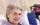Mom is 39 years old. Karl is eight years and Paul 4 years. For many years mother would old just like two children together?
• RT - inscribed circleIn a rectangular triangle has sides lengths> a = 30cm, b = 12.5cm. The right angle is at the vertex C. Calculate the radius of the inscribed circle.
• Semicircle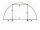To a semicircle with diameter 10 cm inscribe square. What is the length of square sides?
• Find the 9Find the missing angle in the triangle and then name triangle. Angles are: 95, 2x+15, x+3
• The coal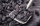The coal stock would be enough to heat a larger room for 12 weeks, a smaller one for 18 weeks. It was heated for four weeks in both rooms, then only in a smaller one. How long was the coal stock enough?Miro stands under a tree and watching its shadow and shadow of the tree. Miro is 180 cm tall and its shade is 1.5 m long. The shadow of the tree is three times as long as Miro's shadow. How tall is the tree in meters?